# Quiz 14: Forecasting Demand for Services

Time series models such as N-period moving average and exponential smoothing methods are used in cases where the values are given in an identifiable pattern. N-moving average method removes the random variations and gives a reliable forecasted value. For given data the forecast of number of patients, staffing and budget levels can be calculated by using the method of N-Period Moving Average. Forecast for patients Annual number of patient test performed: The table given below gives the annual number of patients tests performed: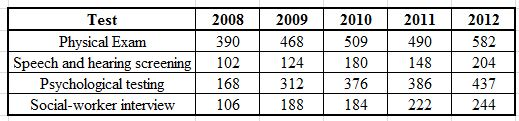A 5-year moving average can be used to calculate the forecast for the number of patients on whom various tests are performed for the year 2013. The moving average formula used in calculating the forecasts is given below: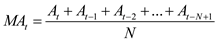…… (1) Thus, put the values of the patients that are tested on physical exam for five years as shown below:Similarly, the 5-year moving average values for the other tests can be calculated by using the formula as given in equation (1). The 5-year moving average values for the other tests is calculated and given in the table as shown below: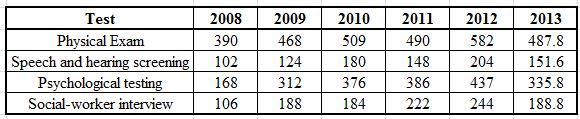Thus, the forecasted values of the 5-year moving average for the tests performed on the patients are given in the table as given above. Monthly patient demand: The monthly patient demand for the period from September 2011-December 2012 is given in the table given below: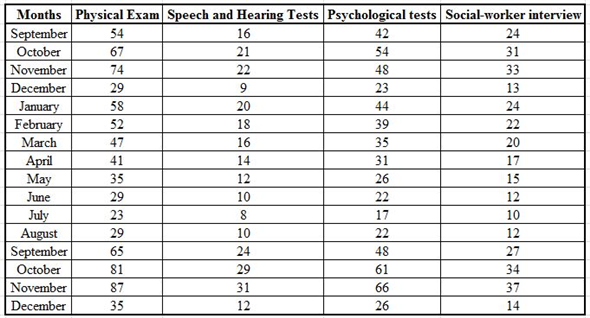The data given above includes seasonal variations as well. A simple moving average method cannot be used in the given case as it would not include the seasonal variations that usually occur in various months. Exponential smoothing with seasonal adjustments is often used in order to counter the variations that arise in various months. The formula for forecasting values using exponential smoothing with seasonal adjustments is given below: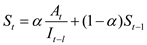In the given formula "I" denotes seasonality index. The value of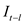denotes seasonality index for the previous year. Here, data is only available from the month of September 2011 onwards. Thus, the value ofcannot be computed which eventually makes it impossible to calculate the forecast values for the month of January 2012 onwards. Budget levels The table given below gives the annual expenses for five years.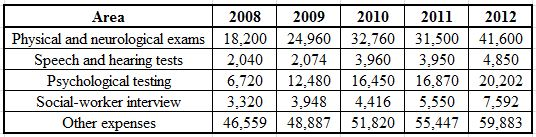By using a 3-year moving average method the forecasted values of various expenses can be calculated. The formula as given below for the moving average method can be used.Annual expenses of physical and neurological exams: The forecasted value of expenses in the area of physical and neurological exams for the year 2013 is calculated as shown below:Similarly, by using a 3-year moving average the annual expenses in the area of speech and hearing tests can also be calculated as shown below:Similarly, the forecast for annual expenses in other areas can also be calculated as shown in the table given below: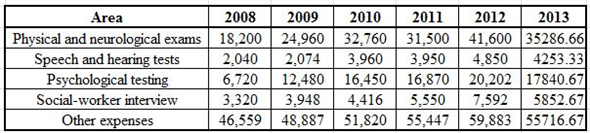Thus, the table given above gives the forecasted values in various areas for the year 2013. Since only the current staffing levels are given and no previous data for staffing levels is provided, no forecast can be made regarding the staffing levels for the next year.
Actual checking-account customers for the month of September were 1,035. The forecasted value for checking-account customers for the month of September made in the month of August were 1,065. Smoothing constant used is 0.1. The forecast for the month of October can be calculated by using simple exponential smoothing formula as given below: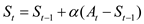…… (1) Where,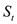is the forecast value for the period t.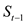is the forecast value for the period t-1.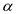is the smoothing constant. Thus, put the given values in equation (1) as shown below:Thus, the forecasted demand for the month of October is 1,062.